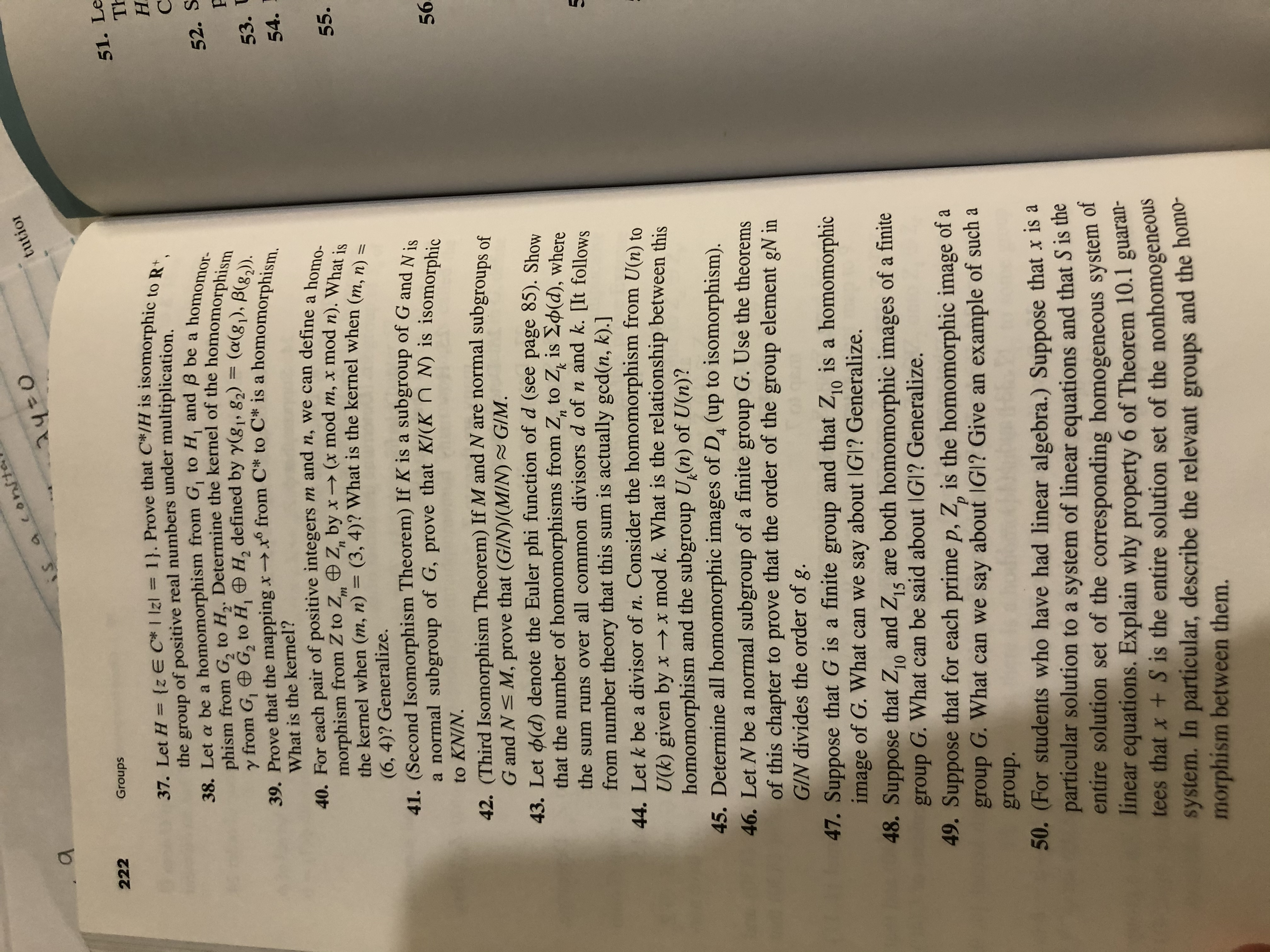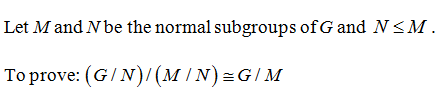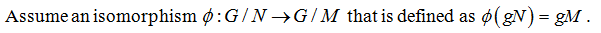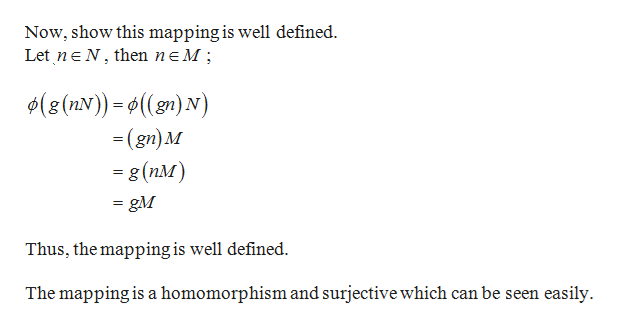# a conitetutior1420iS222Groups51. LeTh37. Let H= {z E C* I Izl 1}. Prove that C*/H is isomorphic to R,of positive real numbers under multiplication.38. Let a be a homomorphism from G, to H, and B be a homomor-phism from G2 to H,. Determine the kernel of the homomorphismfrom G, G2 to H, H, defined by y(g1, 82) = (a(gj), B(82)39. Prove that the mapping xxo from C* to C* is a homomorphism.Н.thegroup52. S53. UWhat is the kernel?40. For each pair of positive integers m and n, we can define a homo-morphism from Z to Z Z, by x- (x mod m, x mod n). What isthe kernel when (m, n) = (3, 4)? What is the kernel when (m, n) =54.т55(6, 4)? Generalize.41. (Second Isomorphism Theorem) If K is a subgroup of G and Nia normal subgroup of G, prove that K/(K N) is isomorphicto KNIN.5642. (Third Isomorphism Theorem) If M and N are normal subgroups ofG and N M, prove that (GIN)/(M/N) G/M.43. Let (d) denote the Euler phi function of d (see page 85). Showthat the number of homomorphisms from Z,, to Z is 2p(d), wherekthe sum runs over all common divisors d of n and k. JIt followsfrom number theory that this sum is actually gcd(n, k).]44. Let k be a divisor of n. Consider the homomorphism from U(n) toU(k) given by x>x mod k. What is the relationship between thishomomorphism and the subgroup U,(n) of U(n)?45. Determine all homomorphic images of D4 (up to isomorphism).46. Let N be a normal subgroup of a finite group G. Use the theoremsof this chapter to prove that the order of the group element gN inGIN divides the order of g.47. Suppose that G is a finite group and that Z10image of G. What can we say about IGI? Generalize.48. Suppose that Z and Z, are both homomorphic images of a finitegroup G. What can be said about IGl? Generalize.49. Suppose that for each prime p, Z, is the homomorphic image ofis a homomorphic1015рgroup G. What can we say about IGI? Give an example of such agroup.50. (For students who have had linear algebra.) Suppose that x S aparticular solution to a system of linear equations and that S is theentire solution set of the corresponding homogeneous system olinear equations. Explain why property 6 of Theorem 10.1 guaran-tees that x + S is the entire solution set of the nonhomogeneoussystem. In particular, describe the relevant groups and the homo-morphism between them.X

Question

42help_outlineImage Transcriptionclosea conite tutior 1420 iS 222 Groups 51. Le Th 37. Let H= {z E C* I Izl 1}. Prove that C*/H is isomorphic to R, of positive real numbers under multiplication. 38. Let a be a homomorphism from G, to H, and B be a homomor- phism from G2 to H,. Determine the kernel of the homomorphism from G, G2 to H, H, defined by y(g1, 82) = (a(gj), B(82) 39. Prove that the mapping xxo from C* to C* is a homomorphism. Н. the group 52. S 53. U What is the kernel? 40. For each pair of positive integers m and n, we can define a homo- morphism from Z to Z Z, by x- (x mod m, x mod n). What is the kernel when (m, n) = (3, 4)? What is the kernel when (m, n) = 54. т 55 (6, 4)? Generalize. 41. (Second Isomorphism Theorem) If K is a subgroup of G and Ni a normal subgroup of G, prove that K/(K N) is isomorphic to KNIN. 56 42. (Third Isomorphism Theorem) If M and N are normal subgroups of G and N M, prove that (GIN)/(M/N) G/M. 43. Let (d) denote the Euler phi function of d (see page 85). Show that the number of homomorphisms from Z,, to Z is 2p(d), where k the sum runs over all common divisors d of n and k. JIt follows from number theory that this sum is actually gcd(n, k).] 44. Let k be a divisor of n. Consider the homomorphism from U(n) to U(k) given by x>x mod k. What is the relationship between this homomorphism and the subgroup U,(n) of U(n)? 45. Determine all homomorphic images of D4 (up to isomorphism). 46. Let N be a normal subgroup of a finite group G. Use the theorems of this chapter to prove that the order of the group element gN in GIN divides the order of g. 47. Suppose that G is a finite group and that Z10 image of G. What can we say about IGI? Generalize. 48. Suppose that Z and Z, are both homomorphic images of a finite group G. What can be said about IGl? Generalize. 49. Suppose that for each prime p, Z, is the homomorphic image of is a homomorphic 10 15 р group G. What can we say about IGI? Give an example of such a group. 50. (For students who have had linear algebra.) Suppose that x S a particular solution to a system of linear equations and that S is the entire solution set of the corresponding homogeneous system o linear equations. Explain why property 6 of Theorem 10.1 guaran- tees that x + S is the entire solution set of the nonhomogeneous system. In particular, describe the relevant groups and the homo- morphism between them. X fullscreen
check_circle

Step 1Step 2...help_outlineImage TranscriptioncloseNow, show this mapping is well defined. Let nE N, then neM (g(nN)-((gn)N) (gn) M =g (nM = gM Thus, the mapping is well defined. The mapping is a homomorphism and surjective which can be seen easily fullscreen

### Want to see the full answer?

See Solution

#### Want to see this answer and more?

Solutions are written by subject experts who are available 24/7. Questions are typically answered within 1 hour.*

See Solution
*Response times may vary by subject and question.
Tagged in

### Math